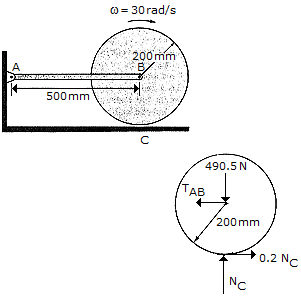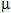Engineering Mechanics - PKRB: Impulse and Momentum - Discussion

Discussion :: PKRB: Impulse and Momentum - General Questions (Q.No.3)

3.The 50-kg cylinder has an angular velocity of 30 rad/s when it is brought into contact with the horizontal surface at C. If the coefficient of friction isc = 0.2, determine how long it takes for the cylinder to stop spinning. What force is developed at the pin A during this time? The axis of the cylinder is connected to two symmetrical links. (Only AB is shown.) For the computation, neglect the weight of the links.

 [A]. t = 1.529 s, A = 0 [B]. t = 3.06 s, A = 0 [C]. t = 1.529 s, A = 49.1 N [D]. t = 3.06 s, A = 49.1 N

Explanation:

No answer description available for this question.